# Waveform¶

Waveform module within the ketos library

This module provides utilities to work with audio data.

Contents:

Waveform class

class `ketos.audio.waveform.``Waveform`(data, time_res=None, filename='', offset=0, label=None, annot=None, transforms=None, transform_log=None, **kwargs)[source]

Audio signal

Args:
rate: float

Sampling rate in Hz

data: numpy array

Audio data

filename: str

Filename of the original audio file, if available (optional)

offset: float

Position within the original audio file, in seconds measured from the start of the file. Defaults to 0 if not specified.

label: int

Spectrogram label. Optional

annot: AnnotationHandler

AnnotationHandler object. Optional

transforms: list(dict)

List of dictionaries, where each dictionary specifies the name of a transformation to be applied to this instance. For example, {“name”:”normalize”, “mean”:0.5, “std”:1.0}

transform_log: list(dict)

List of transforms that have been applied to this instance

Attributes:
rate: float

Sampling rate in Hz

data: 1numpy array

Audio data

time_ax: LinearAxis

Axis object for the time dimension

filename: str

Filename of the original audio file, if available (optional)

offset: float

Position within the original audio file, in seconds measured from the start of the file. Defaults to 0 if not specified.

label: int

Spectrogram label.

annot: AnnotationHandler

AnnotationHandler object.

transform_log: list(dict)

List of transforms that have been applied to this instance

`add`(signal, offset=0, scale=1)[source]

Add the amplitudes of the two audio signals.

The audio signals must have the same sampling rates. The summed signal always has the same length as the present instance. If the audio signals have different lengths and/or a non-zero delay is selected, only the overlap region will be affected by the operation. If the overlap region is empty, the original signal is unchanged.

Args:
signal: Waveform

Audio signal to be added

offset: float

Shift the audio signal by this many seconds

scale: float

Scaling factor applied to signal that is added

Example:
```>>> from ketos.audio.waveform import Waveform
>>> # create a cosine wave
>>> cos = Waveform.cosine(rate=100, frequency=1., duration=4)
>>> # create a morlet wavelet
>>> mor = Waveform.morlet(rate=100, frequency=7., width=0.5)
>>> mor.duration()
3.0
>>> # add the morlet wavelet on top of the cosine, with a shift of 1.5 sec and a scaling factor of 0.5
>>> cos.add(signal=mor, offset=1.5, scale=0.5)
>>> # show the wave form
>>> fig = cos.plot()
>>> fig.savefig("ketos/tests/assets/tmp/morlet_cosine_added.png")
>>> plt.close(fig)
```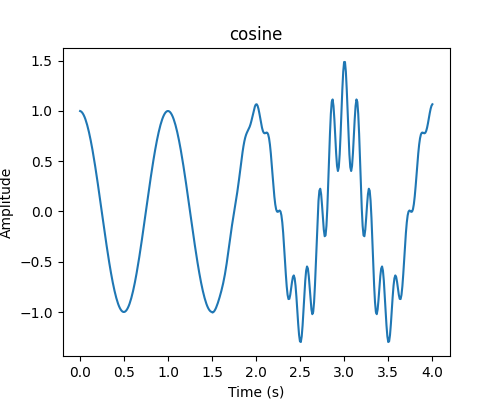`add_gaussian_noise`(sigma)[source]

Add Gaussian noise to the signal

Args:
sigma: float

Standard deviation of the gaussian noise

Example:
```>>> from ketos.audio.waveform import Waveform
>>> # create a morlet wavelet
>>> morlet = Waveform.morlet(rate=100, frequency=2.5, width=1)
>>> morlet_pure = morlet.deepcopy() # make a copy
>>> # add some noise
>>> morlet.add_gaussian_noise(sigma=0.3)
>>> # show the wave form
>>> fig = morlet_pure.plot()
>>> fig.savefig("ketos/tests/assets/tmp/morlet_wo_noise.png")
>>> fig = morlet.plot()
>>> fig.savefig("ketos/tests/assets/tmp/morlet_w_noise.png")
>>> plt.close(fig)
```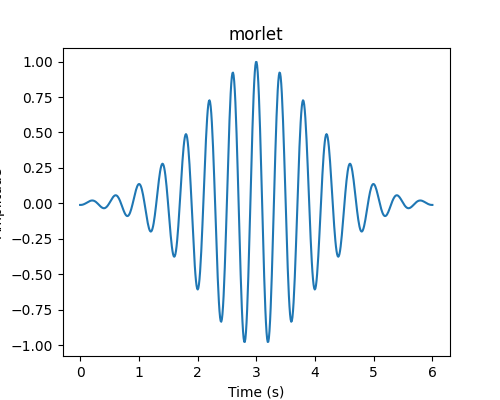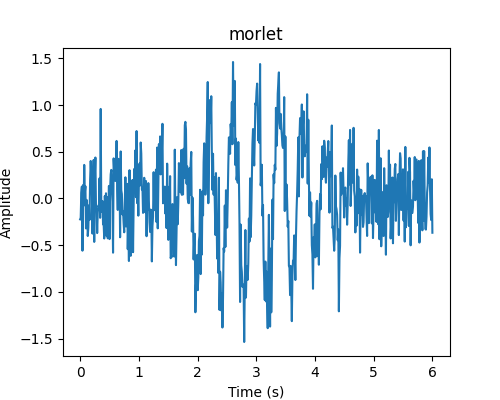`append`(signal, n_smooth=0)[source]

Append another audio signal to the present instance.

The two audio signals must have the same samling rate.

If n_smooth > 0, a smooth transition is made between the two signals in a overlap region of length n_smooth.

Note that the current implementation of the smoothing procedure is quite slow, so it is advisable to use small value for n_smooth.

Args:
signal: Waveform

Audio signal to be appended.

n_smooth: int

Width of the smoothing/overlap region (number of samples).

Returns:

None

Example:
```>>> from ketos.audio.waveform import Waveform
>>> # create a morlet wavelet
>>> mor = Waveform.morlet(rate=100, frequency=5, width=1)
>>> # create a cosine wave
>>> cos = Waveform.cosine(rate=100, frequency=3, duration=4)
>>> # append the cosine wave to the morlet wavelet, using a overlap of 100 bins
>>> mor.append(signal=cos, n_smooth=100)
>>> # show the wave form
>>> fig = mor.plot()
>>> fig.savefig("ketos/tests/assets/tmp/morlet_cosine.png")
>>> plt.close(fig)
```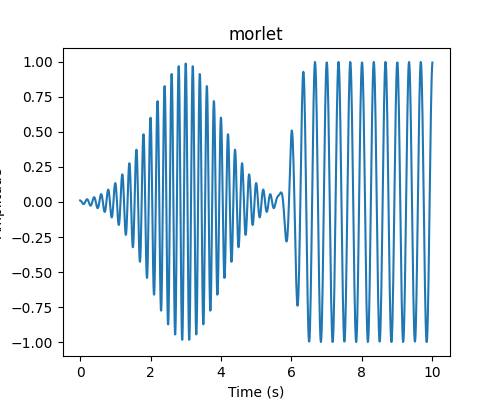classmethod `cosine`(rate, frequency, duration=1, height=1, displacement=0, filename='cosine')[source]

Audio signal with the shape of a cosine function

Args:
rate: float

Sampling rate in Hz

frequency: float

Frequency of the Morlet wavelet in Hz

duration: float

Duration of the signal in seconds

height: float

Peak value of the audio signal

displacement: float

Phase offset in fractions of 2*pi

filename: str

Meta-data string (optional)

Returns:
Instance of Waveform

Audio signal sampling of the cosine function

Examples:
```>>> from ketos.audio.waveform import Waveform
>>> # create a Cosine wave with frequency of 7 Hz
>>> cos = Waveform.cosine(rate=1000., frequency=7.)
>>> # show signal
>>> fig = cos.plot()
>>> fig.savefig("ketos/tests/assets/tmp/cosine_audio.png")
>>> plt.close(fig)
```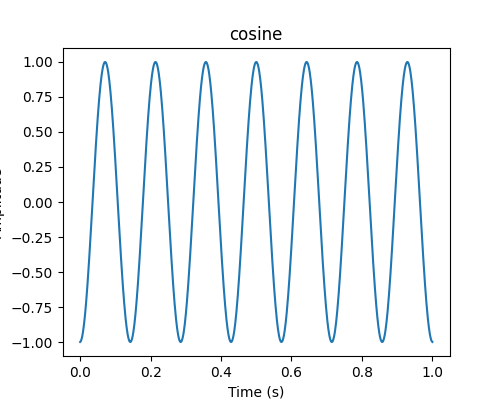classmethod `from_wav`(path, channel=0, rate=None, offset=0, duration=None, resample_method='scipy', id=None, normalize_wav=False, transforms=None, **kwargs)[source]

Load audio data from wave file.

If duration (and offset) are specified and offset + duration exceeds the length of the wav file, the signal will be padded on the right to achieve the desired duration. Similarly, if offset < 0, the signal will be padded on the left. In both cases, a RuntimeWarning is issued.

If offset exceeds the file duration, an empty waveform is returned and a RuntimeWarning is issued.

Args:
path: str

Path to input wave file

channel: int

In the case of stereo recordings, this argument is used to specify which channel to read from. Default is 0.

rate: float

Desired sampling rate in Hz. If None, the original sampling rate will be used

offset: float

Position within the original audio file, in seconds measured from the start of the file. Defaults to 0 if not specified.

duration: float

Length in seconds.

resample_method: str
Resampling method. Only relevant if rate is specified. Options are
• kaiser_best

• kaiser_fast

• scipy (default)

• polyphase

See https://librosa.github.io/librosa/generated/librosa.core.resample.html for details on the individual methods.

id: str

Unique identifier (optional). If None, the filename will be used.

normalize_wav: bool

Normalize the waveform to have a mean of zero (mean=0) and a standard deviation of unity (std=1). Default is False.

transforms: list(dict)

List of dictionaries, where each dictionary specifies the name of a transformation to be applied to this instance. For example, {“name”:”normalize”, “mean”:0.5, “std”:1.0}

Returns:
Instance of Waveform

Audio signal

Example:
```>>> from ketos.audio.waveform import Waveform
>>> # read audio signal from wav file
>>> a = Waveform.from_wav('ketos/tests/assets/grunt1.wav')
>>> # show signal
>>> fig = a.plot()
>>> fig.savefig("ketos/tests/assets/tmp/audio_grunt1.png")
>>> plt.close(fig)
```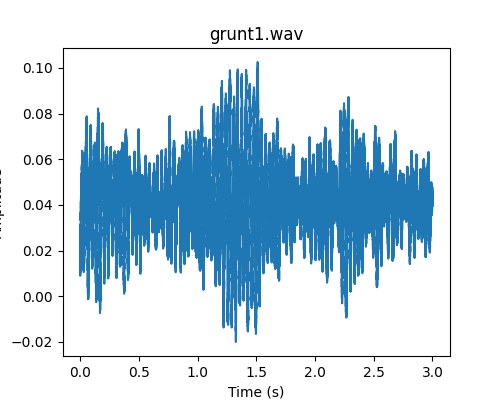classmethod `gaussian_noise`(rate, sigma, samples, filename='gaussian_noise')[source]

Generate Gaussian noise signal

Args:
rate: float

Sampling rate in Hz

sigma: float

Standard deviation of the signal amplitude

samples: int

Length of the audio signal given as the number of samples

filename: str

Meta-data string (optional)

Returns:
Instance of Waveform

Audio signal sampling of Gaussian noise

Example:
```>>> from ketos.audio.waveform import Waveform
>>> # create gaussian noise with sampling rate of 10 Hz, standard deviation of 2.0 and 1000 samples
>>> a = Waveform.gaussian_noise(rate=10, sigma=2.0, samples=1000)
>>> # show signal
>>> fig = a.plot()
>>> fig.savefig("ketos/tests/assets/tmp/audio_noise.png")
>>> plt.close(fig)
```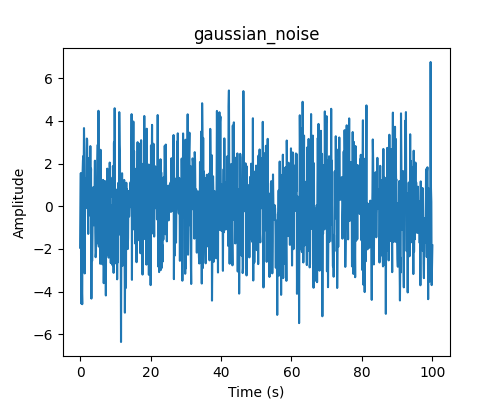`get_attrs`()[source]

Get scalar attributes

`get_data`(id=0)[source]

Get the underlying data numpy array.

Args:
id: int

Audio signal ID. Only relevant if the Waveform object contains multiple, stacked audio signals.

Returns:
d: numpy array

Data

classmethod `morlet`(rate, frequency, width, samples=None, height=1, displacement=0, dfdt=0, filename='morlet')[source]

Audio signal with the shape of the Morlet wavelet

Uses `util.morlet_func()` to compute the Morlet wavelet.

Args:
rate: float

Sampling rate in Hz

frequency: float

Frequency of the Morlet wavelet in Hz

width: float

Width of the Morlet wavelet in seconds (sigma of the Gaussian envelope)

samples: int

Length of the audio signal given as the number of samples (if no value is given, samples = 6 * width * rate)

height: float

Peak value of the audio signal

displacement: float

Peak position in seconds

dfdt: float

Rate of change in frequency as a function of time in Hz per second. If dfdt is non-zero, the frequency is computed as

f = frequency + (time - displacement) * dfdt

filename: str

Meta-data string (optional)

Returns:
Instance of Waveform

Audio signal sampling of the Morlet wavelet

Examples:
```>>> from ketos.audio.waveform import Waveform
>>> # create a Morlet wavelet with frequency of 3 Hz and 1-sigma width of envelope set to 2.0 seconds
>>> wavelet1 = Waveform.morlet(rate=100., frequency=3., width=2.0)
>>> # show signal
>>> fig = wavelet1.plot()
>>> fig.savefig("ketos/tests/assets/tmp/morlet_standard.png")
```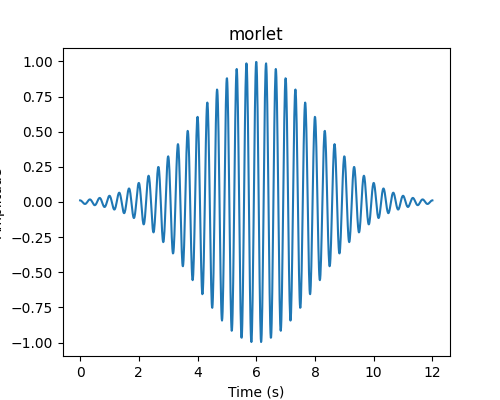```>>> # create another wavelet, but with frequency increasing linearly with time
>>> wavelet2 = Waveform.morlet(rate=100., frequency=3., width=2.0, dfdt=0.3)
>>> # show signal
>>> fig = wavelet2.plot()
>>> fig.savefig("ketos/tests/assets/tmp/morlet_dfdt.png")
>>> plt.close(fig)
```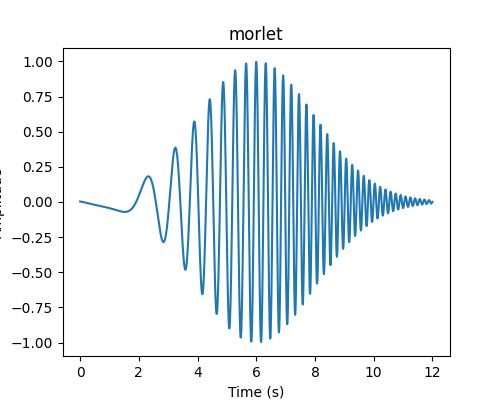`plot`(id=0, show_annot=False)[source]

Plot the data with proper axes ranges and labels.

Optionally, also display annotations as boxes superimposed on the data.

Note: The resulting figure can be shown (fig.show()) or saved (fig.savefig(file_name))

Args:
id: int

ID of data array to be plotted. Only relevant if the object contains multiple, stacked data arrays.

show_annot: bool

Display annotations

Returns:
fig: matplotlib.figure.Figure

Figure object.

Example:
```>>> from ketos.audio.waveform import Waveform
>>> # create a morlet wavelet
>>> a = Waveform.morlet(rate=100, frequency=5, width=1)
>>> # plot the wave form
>>> fig = a.plot()
>>> plt.close(fig)
```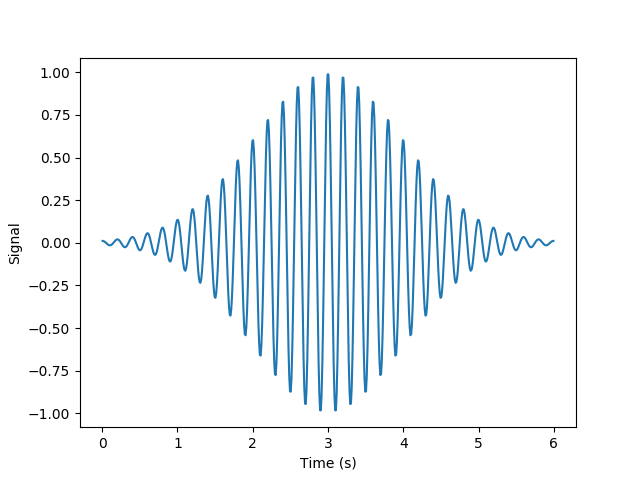`resample`(new_rate)[source]

Resample the acoustic signal with an arbitrary sampling rate.

Note: Code adapted from Kahl et al. (2017)
Args:
new_rate: int

New sampling rate in Hz

`segment`(window, step=None)[source]

Divide the time axis into segments of uniform length, which may or may not be overlapping.

Window length and step size are converted to the nearest integer number of time steps.

If necessary, the audio signal will be padded with zeros at the end to ensure that all segments have an equal number of samples.

Args:
window: float

Length of each segment in seconds.

step: float

Step size in seconds.

Returns:
segs: Waveform

Stacked audio signals

Example:
```>>> from ketos.audio.waveform import Waveform
>>> # create a morlet wavelet
>>> mor = Waveform.morlet(rate=100, frequency=5, width=0.5)
>>> mor.duration()
3.0
>>> # segment into 2-s wide frames, using a step size of 1 s
>>> segs = mor.segment(window=2., step=1.)
>>> # show the segments
>>> fig0 = segs.plot(0)
>>> fig0.savefig("ketos/tests/assets/tmp/morlet_segmented_0.png")
>>> fig1 = segs.plot(1)
>>> fig1.savefig("ketos/tests/assets/tmp/morlet_segmented_1.png")
>>> plt.close(fig0)
>>> plt.close(fig1)
````to_wav`(path, auto_loudness=True)[source]

Save audio signal to wave file

Args:
path: str

Path to output wave file

auto_loudness: bool

Automatically amplify the signal so that the maximum amplitude matches the full range of a 16-bit wav file (32760)